# Daniel Soudry - Deep Learning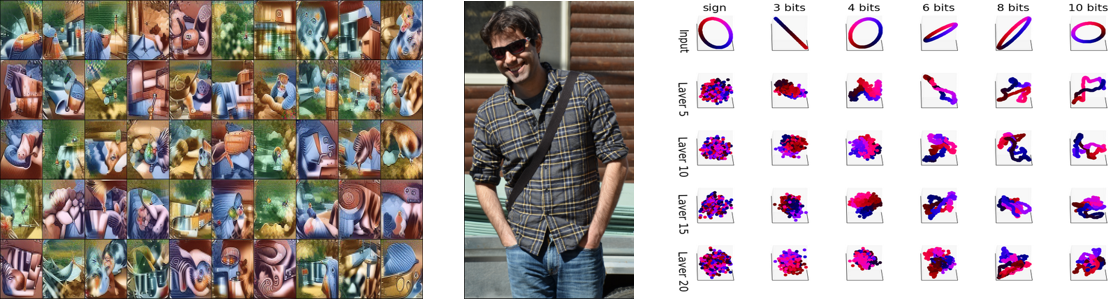## Machine Learning

There are several open theoretical questions in deep learning. Answering these theoretical questions will provide design guidelines and help with some important practicals issue (explained below). Two central questions are:

• Low training error. Neural Networks are often initialized randomly, and then optimized using local steps with stochastic gradient descent (SGD). Surprisingly, we often observe that SGD converges to a low training error: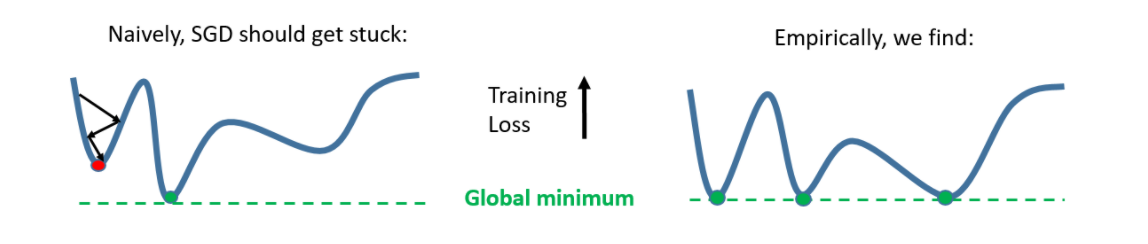Why is this happening?

• Low generalization error. Neural networks are often trained in a regime where #parameters » #data samples. Surprisingly, these networks generalize well in such a regime, even when there is no explicit regularization. For example, as can be seen in the figure below (from Wu, Zu & E 2017), polyomial curves (right) tend to overfit much more than neural networks (left):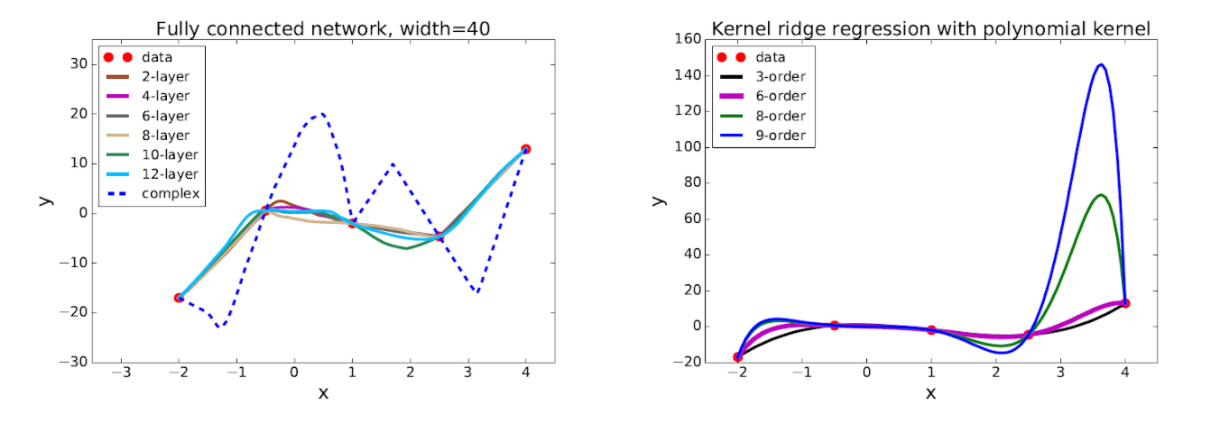Why is this happening?

There are many practical bottlenecks in deep learning (the following figures are from Sun et al. 2017). Such bottlenecks occur since neural networks models are large, and keep getting larger over the years: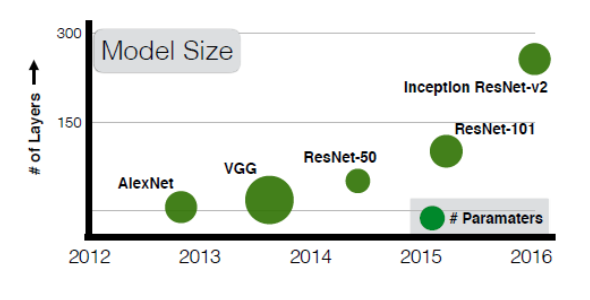• Computational resources. Using larger neural networks require more computational resources, such as power-hungry GPUs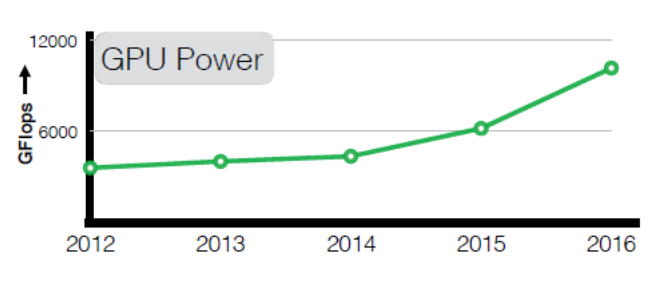How can we train and use neural networks more efficiently (i.e., better speed, energy, memory), without sacrificing accuracy? See my talk here (in Hebrew) for some of our results on this.

• Labeled data. In order to train neural nets to high accuracy levels, large quantities of labeled data are required. Such datasets are hard to obtain. For example, for many years the size of the largest vision training data remained constant:How can we decrease the amount of label data required for training?

• Choosing hyper-parameters. Since larger models take longer to train, it becomes more challenging to choose model hyper-parameters (e.g., architecture, learning rate) in order to obtain good performance. For example, as can be seen in this video (Xian&Li 2017), small changes in the training procedure have a large effect on the network performance:

Can we find automatic and robust method to find the "optimal" hyper-parameters?